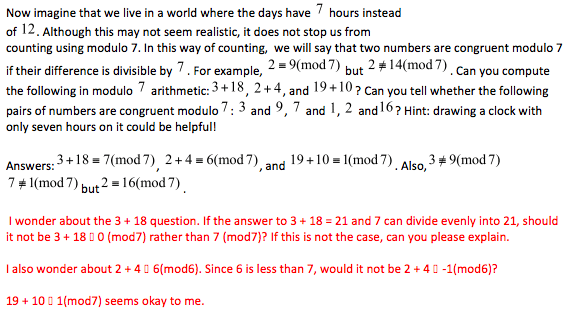SEARCH HOMEMath Central Quandaries & QueriesHello, I am editing a resource for students, and I think some of the answers may be incorrect. The text I was given and my questions are in the attachment. Any help you could give would be appreciated. Thanks, KimHere is what Kim sent.Hi Kim,

In the next to the last line of what you sent you have mod6, I assume that is a typo and should be mod7.

One of the challenges of working with modular arithmetic is that a question might have many different, correct answers. For example in your test is the true statement that $2 \equiv 9$(mod 7) but it is also true that $2 \equiv 16$(mod 7), $2 \equiv 23$(mod 7) and $2 \equiv -5$(mod 7). In fact 2 is equivalent to 2 plus or minus any multiple of 7, modulo 7. Hence the statement in the text that $3 + 18 \equiv 7$(mod 7) and your statement that $3 + 18 \equiv 0$(mod 7) are both true.

It is conventional that when performing a calculation modulo 7, the answer be given as a number between 0 and 6 inclusive. Hence I would prefer your answer that $3 + 18 \equiv 0$(mod 7). For the same reason I would prefer the answer in the text that states $2 + 4 \equiv 6$(mod 7) to your, nevertheless true statement, that $2 + 4 \equiv -1$(mod 7).

HarleyMath Central is supported by the University of Regina and The Pacific Institute for the Mathematical Sciences.